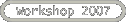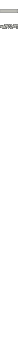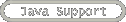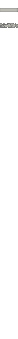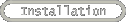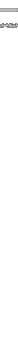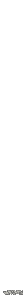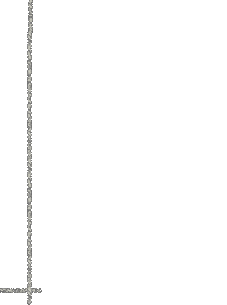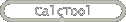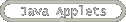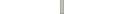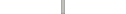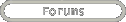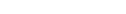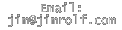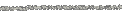# History

### Version 1.6

1. Fixed bug occuring when calculating line integrals.

### Version 1.4

1. Cleaned up look by adding different axes style options. Default is crossed axes, xy plane, and xy grid.
2. Fixed some transparency issues.
3. Fixed but in dot/cross tab where rotating vector inadvertently increased the length.
4. Fixed bug on Vector Field tab where changing # grid points did not correctly change distance between each vector.
5. Using domain/range menu did not correctly re-graph surface. This has been fixed.
6. Added ability on deriv tab to change min/max values for theta.
7. Fixed some issues with syncing/unsyncing domain of contour map and domain of 3d surface.

### Version 1.0

1. Version 1.0 designation.
2. Colors of some objects have been changed.
3. Option to specify number of grid points on vector field has been added.

### Version 0.8

1. Contours tab has been made functional. This includes graphing contours of a surface, the gradient at a point (and associated contour), as well as the option to graph a constraint equation as well.
2. Some labels were chopped off in on Windows platform; this has been fixed.
3. Symbolic calculation of divergence and curl on vector field panel has been added.
4. Domain panel on Deriv panel is now hidded as default.
5. Circular domain panel has been slightly reformatted.
6. "Net Area" is now invisible as default on Int panel for line integrals.

### Version 0.6

1. Added "Deriv" panel. This provides the ability to visualize slices of surfaces that yield curves that can be used to visualize directional derivatives.
2. Symbolic computation of partial derivatives is provided.
4. The ability to change the direction of slices (and hence the direction of the derivative) is added via a slider.

### Version 0.5.5

1. Acceleration vector was not being computed in certain cases. This has been fixed.

### Version 0.5

1. Added ability to graph both tangent and unit tangent vectors on parametric curves.
2. Added ability to graph both acceleration vector on parametric curves.

### Version 0.4

1. Added slider to parametric curve graph to move tangent/normal/binormal vectors interactively.
2. Added ability to graph up to 10 points, and connect these points with a line, and/or form a closed loop.
3. Added ability to graph rectangular surfaces of form y=g(x,z) and x=h(y,z).
4. Added circular domain option for rectangular surfaces.
5. Parametric curves did not graph the max value for "t". This has been fixed.

### Version 0.3

1. Added Dot/Cross tab to compute and visualize dot products and cross products.
2. Fixed bug where vectors parallel to (0,0,-1) would not graph correctly.

### Version 0.2

1. Added option of graphing xy plane.
2. Added functionality to all tangent/normal/binormal vector boxes.
3. Changed layout slightly.

### Version 0.1

1. First version with basic functionality. User can graph points, vectors, parametric surfaces, parametric curves, and rectangular surfaces in 3D.